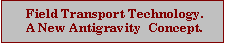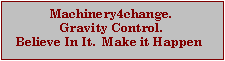Acceleration And Velocity
It can be quite confusing applying the formula for planetary gravity to the likely velocity achievable by using a continuously accelerating machine. Finding examples of this is not easy, and so far I have found only two. Both estimate velocities for travel to the Moon. Petar Bosnic Petrus, who claims to have built an experimental machine is one,  and Leonard Cramp is the other.
I have chosen to use a computer program (dBase 111+) to calculate the velocities and distances at 1 second increments, shown on the screen in real time, and to store the results in a database for transfer to a spreadsheet program to create a graph. Shown below is a graph which uses the formula   9.8m/sec/sec with a time span of 540 seconds.

The magenta line is the total distance travelled  which is about 400,000 km.
The blue line is the average velocity over 540 sec which is about 3.4 million KPH
The yellow line is the velocity at each sec. At 540 sec it is about 10.2 million KPH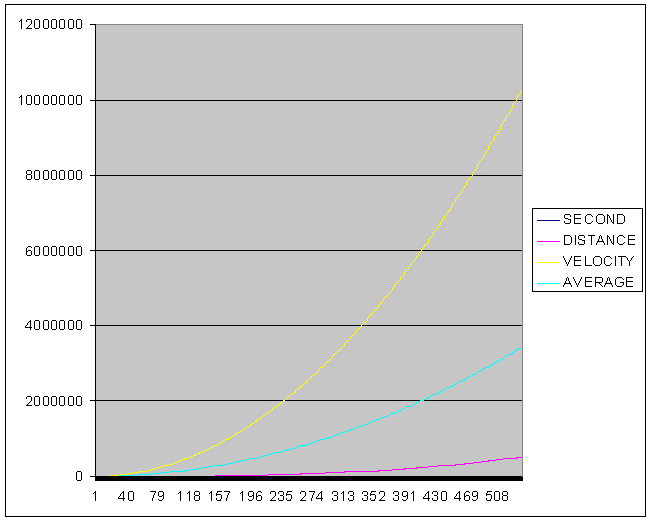Going past the Moon  at 8.5 million KPH in just 8.2 minutes.
Samples below are from the first and last 50 seconds of the program run.
KPH          KPH            SEC        DIST          VEL KPH    AV KPH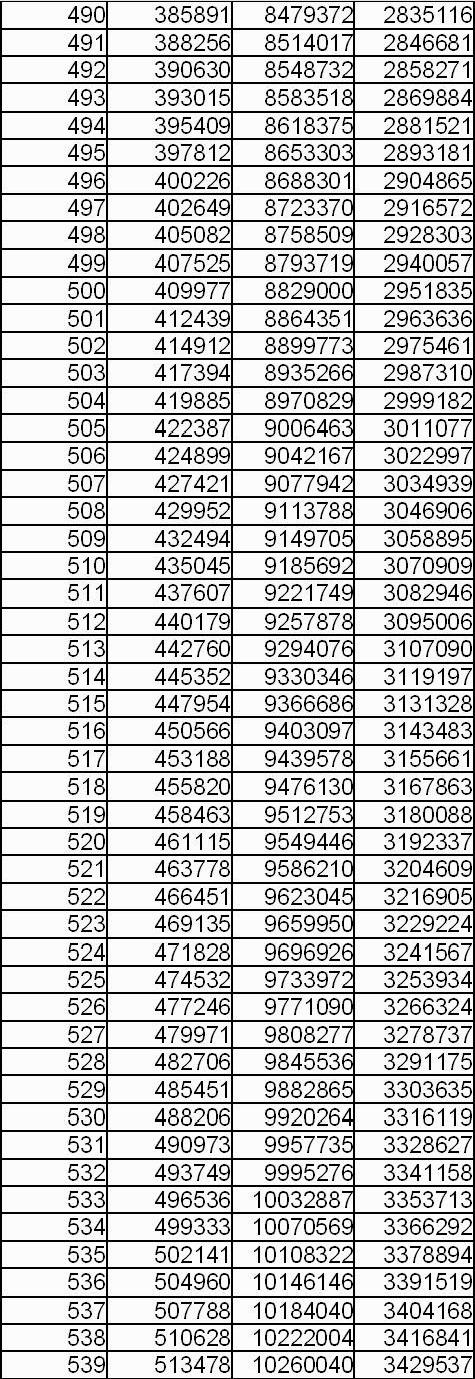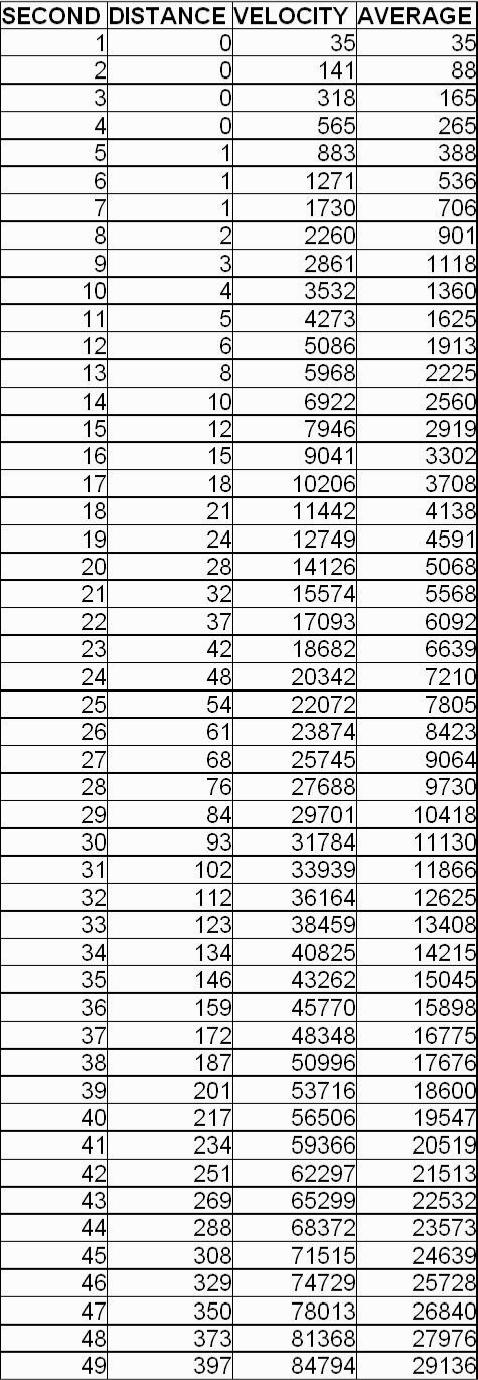The formula for gravity is 9.8 m/sec/sec. My interpretation of how to calculate continuous acceleration away from the Earth is incorporated into the dBase 111+ program above, and is as follows.

During second 1 of acceleration, starting from 0 velocity @ 9.8 * 1 * 1 = 9.8 meters travelled. The velocity is now 0.0098 x 3600 = 35.28 KPH.

During second 2 of acceleration, starting from 35.28KPH velocity @ 9.8 * 2 * 2 = 39.2 meters travelled and the velocity has increased by 0.0392 * 3600 = 141.12 KPH.

At the end of second 2 the velocity is 35.28 + 141.12 = 176.4 KPH and the total distance travelled is 9.8 + 39.2 = 49 meters.

During second 3 of acceleration, starting from 176.4 KPH velocity @ 9.8 * 3 * 3 = 88.2
meters travelled and the velocity has increased by 0.0882 * 3600 = 317.52 KPH.

At the end of second 3 the velocity is 176.4 + 317.52 = 493.92 KPH and the total distance travelled is 49 + 88.2 = 137.2 meters.

And so on. The effect of air resistance may be a slowing factor, but this would reduce rapidly as the atmosphere thins. The effect of gravity would reduce exponentially  as a machine moves away from the Earth.

All the above calculations are for 1g acceleration. But 1g would be enough to hover only.
Any amount over 1 would create acceleration, eg 1.1g would provide 0.1g.